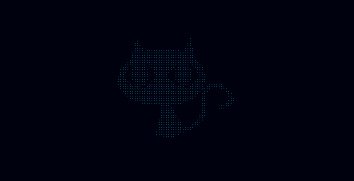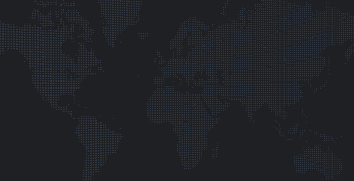Ola everyone !

I always wanted to write articles on CodePen. Here is my first one !
Every comment is welcome :)

For a few pens I used a technique I wanted to share. I really like particles and I found interesting to create them from an image.

#### Deep cat#### Dotted mapIn this tutorial I will just explain how to generate an array filled with coordinates from the pixels of our image. Those pens above are using ThreeJs to create a depth effect but I won't get into 3D over here. I will just use the Canvas API ;)

### What do we need to start ?

• You need a basic canvas tag in your HTML
```  ```<canvas id="scene"></canvas>
```
```
• We will use some CSS to make our result nicer
```  ```body, html{
width:100%;
height:100%;
overflow: hidden;
background: black;
}
canvas{
position: absolute;
top:50%;
left:50%;
transform: translate(-50%,-50%);
}
```
```
• And finally you need your imageBe careful with the size of your image. I'm using a 100x100px size which is already a huge amount of pixels (10 000 !!)

### Get the infos of your image

Now that we have our basic scene we need to convert our image into an array of data.

• Create a new image element and execute the 'drawScene' function when it is loaded.
```  ```var png = new Image();
png.src = "codepen.png";
```
```
• Now that our image is loaded, we can init our canvas scene.
```  ```var canvas    = document.getElementById("scene");
var ctx       = canvas.getContext("2d");

canvas.width = png.width;
canvas.height = png.height;
```
```
• The next step is to draw the image, get the data from the scene and finally clearing it. To get the data we will use the `getImageData` method from the Canvas API.
This method returns an object with an array in it that contains 4 values for each pixel: one for each color (RGB) and a last one for the Alpha.
```  ```ctx.drawImage(png, 0, 0);
var data = ctx.getImageData(0, 0, png.width, png.height);
ctx.clearRect(0,0,canvas.width, canvas.height);
```
```
• We now have an array with the data of every pixel from the image. But we only want specific pixels. In this case I will select only the pixel with no transparency (but you can target all the blue pixels, the darker pixels [...] It's up to you !). To select the pixels we need, we will loop through the Y and the X axis of our image. That's why we have a loop into another one.
I check if it's four value (Alpha) is over than 128, the average value. (Each value is between 0 and 255).
If the Alpha is over 128, I push the pixel into my `particles` array.
```  ```var particles = [];
for (var y = 0, y2 = data.height; y < y2; y++) {
for (var x = 0, x2 = data.width; x < x2; x++) {
if (data.data[(x * 4 + y * 4 * data.width) + 3] > 128) {
var particle = {
x : x,
y : y
};
particles.push(particle);
}
}
}
```
```

### Check if the array is correct

We can do a quick check by drawing every particle on our scene.

1. Set the fillStyle to white.
2. Loop through all the particles.
3. Draw each particle with its coordinates.
4. And voila !
```  ```ctx.fillStyle = "white";
for(var i=0, j=particles.length;i<j;i++){
var particle = particles[i];
ctx.fillRect(particle.x, particle.y, 1, 1);
}
```
```

For the demos I had to encode my png into a Base64 image because of cross-domain issue.